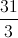Example Questions

← Previous 1 3

Example Question #1 : How To Find X Or Y Intercept

If the equation of a line is 4y – x = 48, at what point does that line cross the x-axis?

(0,–12)

(0,12)

(48,0)

(0,–48)

(–48,0)

(–48,0)

Explanation:

When the equation crosses the x-axis, y = 0.  Plug 0 into the equation for y, and solve for x

4(0) – x = 48,  –x = 48,  x = –48

Example Question #2 : How To Find X Or Y Intercept

The slope of a line is equal to -3/4.  If that line intersects the y-axis at (0,15), at what point does it intersect the x-axis?

15

-20

60

5

20

20

Explanation:

If the slope of the line m=-3/4, when y=15 and x=0, plug everything into the equation y=mx+b.

Solving for b:

15=(-3/4)*0 + b

b=15

y=-3/4x + 15

To get the x-axis intersect, plug in y=0 and solve for x.

0 = -3/4x + 15

3/4x = 15

3x = 15*4

x = 60/3 = 20

x=20

Example Question #1 : How To Find X Or Y Intercept

If these three points are on a single line, what is the formula for the line?

(3,3)

(4,7)

(5,11)

y = 3x - 3

y = 4x + 31

y = 5x + 11

y = 4x - 9

y = 3x - 9

y = 4x - 9

Explanation:

Formula for a line: y = mx + b

First find slope from two of the points: (3,3) and (4,7)

m = slope = (y2 – y1) / x2 – x1) = (7-3) / (4-3) = 4 / 1 = 4

Solve for b by plugging m and one set of coordinates into the formula for a line:

y = mx + b

11 = 4 * 5 + b

11 = 20 + b

b = -9

y = 4x - 9

Example Question #4 : How To Find X Or Y Intercept

The slope of a line is 5/8 and the x-intercept is 16.  Which of these points is on the line?

(16, 10)

(0,10)

(8,15)

(32,10)

(32,30)

(32,10)

Explanation:

y = mx + b

x intercept is 16 therefore one coordinate is (16,0)

0 = 5/8 * 16 + b

0 = 10 + b

b = -10

y = 5/8 x – 10

if x = 32

y = 5/8 * 32 – 10 = 20 – 10 = 10

Therefore (32,10)

Example Question #5 : How To Find X Or Y Intercept

A line has the equation: x+y=1.

What is the y-intercept?

0.5

2

1

-1

0

1

Explanation:

x+y=1 can be rearranged into: y=-x+1. Using the point-slope form, we can see that the y-intercept is 1.

Example Question #6 : How To Find X Or Y Intercept

A line has the equation: 2x+4y=8.

What is the x-intercept?

4

-4

8

-8

0

4

Explanation:

To find the x-intercept, rearrange the equation 2x+4y=8 so that x is isolated:

2x=-4y+8

x=-2y+4

Using the point-slope formula, we see that the x-intercept is 4.

Example Question #7 : How To Find X Or Y Intercept

What is the y intercept of the following function of x?

y = 3x

3

–3

0

1

–1

0

Explanation:

The answer is 0 because in slope intercept form, y = mx + b; b is the y intercept. In this case b = 0.

Example Question #8 : How To Find X Or Y Intercept

What is the x-intercept of a line with a slope of 5 and y-intercept of 3.5?

(–0.7, 0)

(3.5, 0)

(0.7, 0)

(–3.5, 0)

(0, –0.7)

(–0.7, 0)

Explanation:

To solve this, first find the equation of our line.  The form of the question gives it to us very directly.  We can use the slope-intercept form (y = mx + b).

y = 5x + 3.5

The x-intercept is found by setting y = 0, because that will give us the x-value at which the line crosses the x-axis.

0 = 5x + 3.5; –3.5 = 5x; x = –3.5 / 5 or –0.7.  The point will be (–0.7, 0)

Example Question #9 : How To Find X Or Y Intercept

Determine the y-intercept of the following line: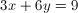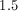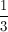Explanation:

The y-intercept occurs when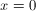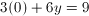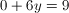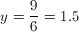Example Question #10 : How To Find X Or Y Intercept

At what point does the graph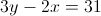cross the-axis?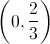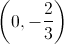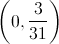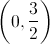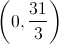The graph crosses the-axis where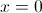. So plugging in and solving yields